## RS Aggarwal Class 8 Solutions Chapter 4 Cubes and Cube Roots Ex 4D

These Solutions are part of RS Aggarwal Solutions Class 8. Here we have given RS Aggarwal Solutions Class 8 Chapter 4 Cubes and Cube Roots Ex 4D.

Other Exercises

Objective Questions
Tick the correct answer in each of the following:

Question 1.
Solution:
(a) 141
= 3 x 47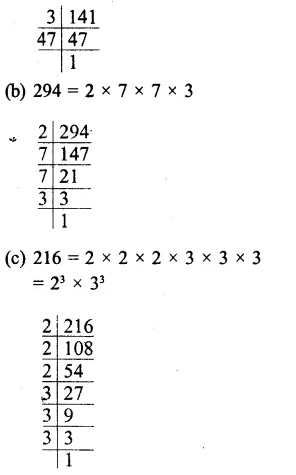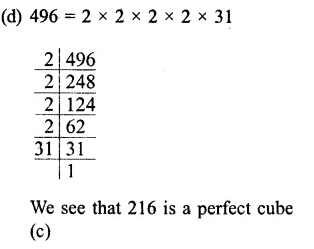Question 2.
Solution:
1152
= 2 x 2 x 2 x 2 x 2 x 2 x 3 x 3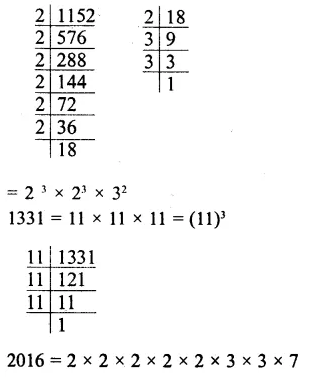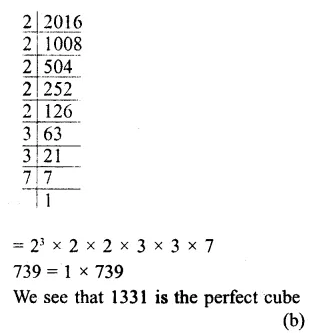Question 3.
Solution:
$$\sqrt [ 3 ]{ 512 }$$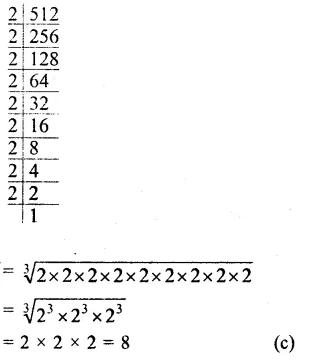Question 4.
Solution:
$$\sqrt [ 3 ]{ 125\times 64 }$$Question 5.
Solution:
$$\sqrt [ 3 ]{ \frac { 64 }{ 343 } }$$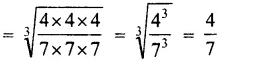Question 6.
Solution:
$$\sqrt [ 3 ]{ \frac { -512 }{ 729 } }$$Question 7.
Solution:
Factorising 648, we get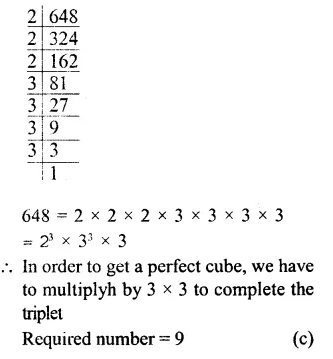Question 8.
Solution:
Factorising 1536, we get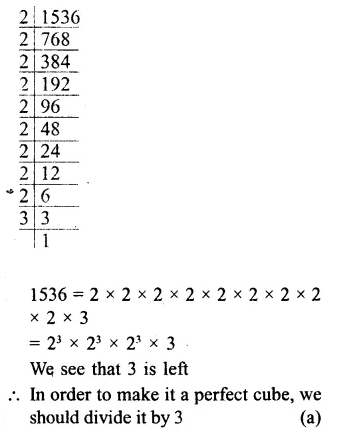Question 9.
Solution:
$${ \left( 1\frac { 3 }{ 10 } \right) }^{ 3 }$$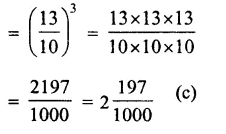Question 10.
Solution:
(0.8)³
= 0.8 x 0.8 x 0.8 = 0.512 (c)

Hope given RS Aggarwal Solutions Class 8 Chapter 4 Cubes and Cube Roots Ex 4D are helpful to complete your math homework.

If you have any doubts, please comment below. Learn Insta try to provide online math tutoring for you.